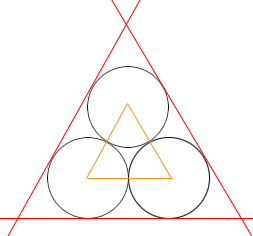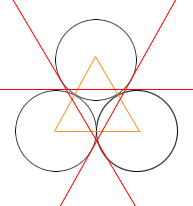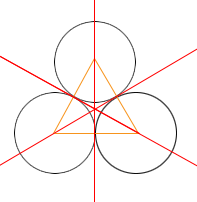SEARCH HOMEMath Central Quandaries & QueriesQuestion from tushar, a student: draw an equilateral triangle with side 6cm.draw 3circles with radii 3cm on each angular point of triangle.draw common tangent on each of two circlesTushar,

In my diagrams the starting equilateral is yellow and the circles are black.In the diagram above each of the red lines is tangent to two of the circles.In the diagram above each of the red lines is tangent to two of the circles.In the diagram above each of the red lines is tangent to two of the circles.

I am not sure what diagram you want as you didn't ask a question.

PennyMath Central is supported by the University of Regina and The Pacific Institute for the Mathematical Sciences.